# Shift Arithmetic

Shift bits or binary point of signal

## Library

Logic and Bit Operations

•## Description

### Supported Shift Operations

The Shift Arithmetic block can shift the bits or the binary point of an input signal, or both.

For example, shifting the binary point on an input of data type `sfix(8)`, by two places to the right and left, gives these decimal values.

Shift OperationBinary ValueDecimal Value

No shift (original number)

11001.011

–6.625

Binary point shift right by two places

1100101.1

–26.5

Binary point shift left by two places

110.01011

–1.65625

This block performs arithmetic bit shifts on signed numbers. Therefore, the block recycles the most significant bit for each bit shift. Shifting the bits on an input of data type `sfix(8)`, by two places to the right and left, gives these decimal values.

Shift OperationBinary ValueDecimal Value

No shift (original number)

11001.011

–6.625

Bit shift right by two places

11110.010

–1.75

Bit shift left by two places

00101.100

5.5

## Data Type Support

The block supports input signals of the following data types:

Input SignalSupported Data Types
`u`
• Floating point

• Built-in integer

• Fixed point

`s`
• Floating point

• Built-in integer

• Fixed-point integer

The following rules determine the output data type:

Data Type of Input uOutput Data Type
Floating pointSame as input `u`
Built-in integer or fixed point
• Sign of `u`

• Word length of `u`

• Slope of `u * 2^(max(binary points to shift))`

• Bias of ```u * 2^(max(binary points to shift – bits to shift))``` , for bit shifts where the direction is bidirectional or right

• Bias of ```u * 2^(max(binary points to shift + bits to shift))```, for bit shifts where the direction is left

The block parameters support the following data types:

ParameterSupported Data Types
Bits to shift: Number
• Built-in integer

• Fixed-point integer

Binary points to shift
• Built-in integer

• Fixed-point integer

## Parameters

Bits to shift: Source

Specify whether to enter the bits to shift on the dialog box or to inherit the values from an input port.

Bits to shift: Direction

Specify the direction in which to shift bits: left, right, or bidirectional.

Bits to shift: Number

Specify a scalar, vector, or array of bit shift values. This parameter is available when Bits to shift: Source is `Dialog`.

If the direction is...Then...
`Left` or `Right`Use positive integers to specify bit shifts.
`Bidirectional`Use positive integers for right shifts and negative integers for left shifts.
Binary points to shift

Specify an integer number of places to shift the binary point of the input signal. A positive value indicates a right shift, while a negative value indicates a left shift.

Diagnostic for out-of-range shift value

Specify whether to produce a warning or error during simulation when the block contains an out-of-range shift value. Options include:

• `None` — Simulink® software takes no action.

• `Warning` — Simulink software displays a warning and continues the simulation.

• `Error` — Simulink software terminates the simulation and displays an error

For more information, see Simulation and Accelerator Mode Results for Out-of-Range Bit Shift Values.

Check for out-of-range 'Bits to shift' in generated code

Select this check box to include conditional statements in the generated code that protect against out-of-range bit shift values. This check box is available when Bits to shift: Source is ```Input port```.

## Out-of-Range Bit Shifts

### Definition of an Out-of-Range Bit Shift

Suppose that `WL` is the input word length. The shaded regions in the following diagram show out-of-range bit shift values for left and right shifts.Similarly, the shaded regions in the following diagram show out-of-range bit shift values for bidirectional shifts.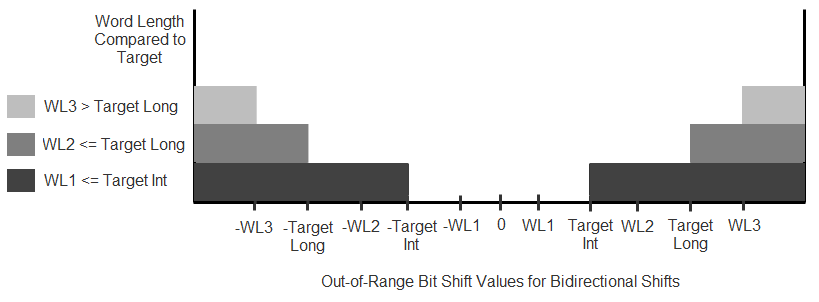The diagnostic for out-of-range bit shifts responds as follows, depending on the mode of operation:

ModeDiagnostic for out-of-range shift value
NoneWarningError
Simulation modesDo not report any warning or error.Report a warning but continue simulation.Report an error and stop simulation.
Code generationHas no effect.

### Simulation and Accelerator Mode Results for Out-of-Range Bit Shift Values

Suppose that `U` is the input, `WL` is the input word length, and `Y` is the output. The output for an out-of-range bit shift value for left shifts is as follows: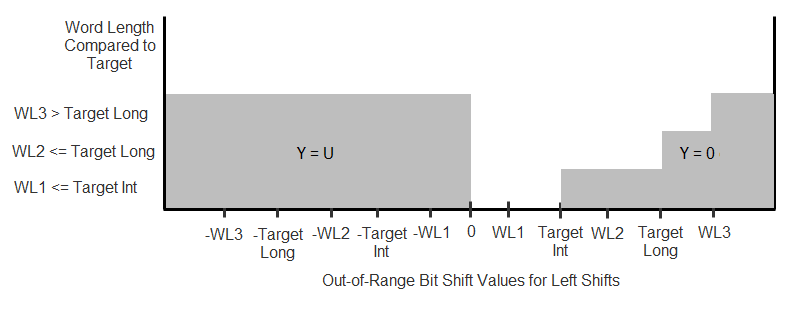Similarly, the output for an out-of-range bit shift value for right shifts is as follows: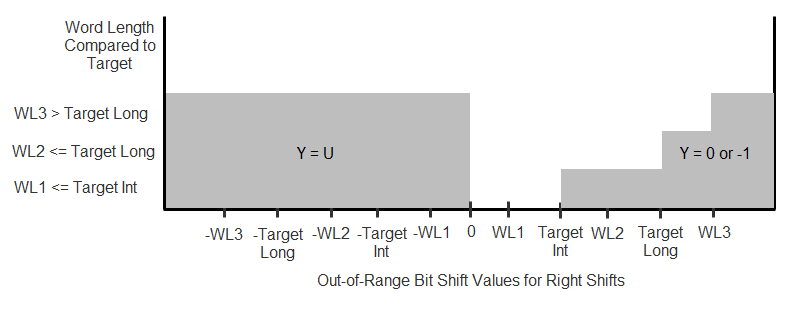For bidirectional shifts, the output for an out-of-range bit shift value is as follows: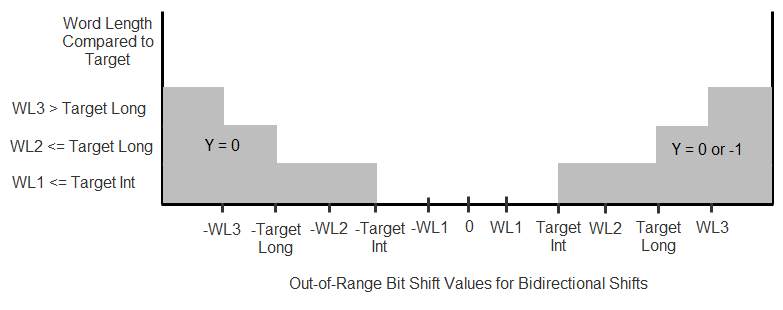### Code Generation for Out-of-Range Bit Shift Values

For the generated code, the method for handling out-of-range bit shifts depends on the setting of Check for out-of-range 'Bits to shift' in generated code.

Check Box SettingGenerated CodeSimulation Results Compared to Generated Code

Selected

Includes conditional statements to protect against out-of-range bit shift values.

Simulation and Accelerator mode results match those of code generation.

Cleared

Does not protect against out-of-range bit shift values.

• For in-range values, simulation and Accelerator mode results match those of code generation.

• For out-of-range values, the code generation results are compiler specific.

For right shifts on signed negative inputs, most C compilers use an arithmetic shift instead of a logical shift. Generated code for the Shift Arithmetic block depends on this compiler behavior.

## Examples

### Block Output for Right Bit Shifts

The following model compares the behavior of right bit shifts using the dialog box versus the block input port.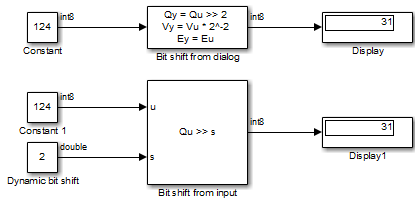The key block parameter settings of the Constant blocks are:

BlockParameterSetting
`Constant` and `Constant1`Constant value`124`
Output data type`int8`
`Dynamic bit shift`Constant value`2`
Output data type`Inherit: Inherit from 'Constant value'`

The key block parameter settings of the Shift Arithmetic blocks are:

BlockParameterSetting
`Bit shift from dialog`Bits to shift: Source`Dialog`
Bits to shift: Direction`Right`
Bits to shift: Number`2`
`Bit shift from input`Bits to shift: Source`Input port`
Bits to shift: Direction`Right`

The top Shift Arithmetic block takes an input of 124, which corresponds to 01111100 in binary format. Shifting the number of bits two places to the right produces 00011111 in binary format. Therefore, the block outputs 31.

The bottom Shift Arithmetic block performs the same operation as the top block. However, the bottom block receives the bit shift value through an input port instead of the dialog box. By supplying this value as an input signal, you can change the number of bits to shift during simulation.

### Block Output for Binary Point Shifts

The following model shows the effect of binary point shifts.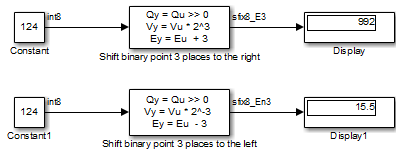The key block parameter settings of the Constant blocks are:

BlockParameterSetting
`Constant` and `Constant1`Constant value`124`
Output data type`int8`

The key block parameter settings of the Shift Arithmetic blocks are:

BlockParameterSetting
`Shift binary point 3 places to the right`Bits to shift: Source`Dialog`
Bits to shift: Direction`Bidirectional`
Bits to shift: Number`0`
Binary points to shift: Number`3`
`Shift binary point 3 places to the left`Bits to shift: Source`Dialog`
Bits to shift: Direction`Bidirectional`
Bits to shift: Number`0`
Binary points to shift: Number`3`

The top Shift Arithmetic block takes an input of 124, which corresponds to 01111100 in binary format. Shifting the binary point three places to the right produces 01111100000 in binary format. Therefore, the top block outputs 995.

The bottom Shift Arithmetic block also takes an input of 124. Shifting the binary point three places to the left produces 01111.100 in binary format. Therefore, the bottom block outputs 15.5.

## Characteristics

 Data Types Double | Single | Base Integer | Fixed-Point Sample Time Inherited from driving block Direct Feedthrough Yes Multidimensional Signals No Variable-Size Signals No Zero-Crossing Detection No Code Generation Yes

## Extended Capabilities

### Fixed-Point ConversionDesign and simulate fixed-point systems using Fixed-Point Designer™.

Introduced before R2006a